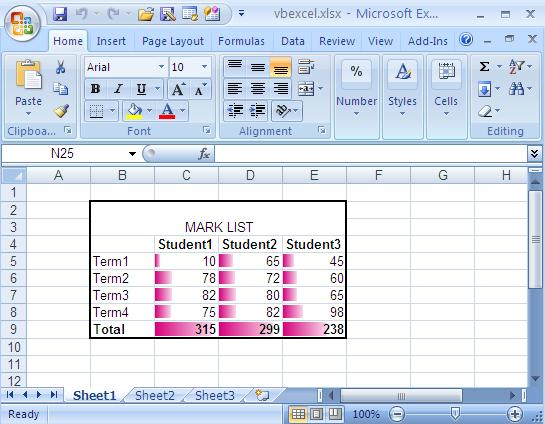Programming Tutorials

Using Excel 2007 DataBar in VB.net

By: Issac Printer Friendly Format

This tutorial helps you o construct a data bar inside an Excel 2007 sheet, here we enter the data of a Mark List and format the page. Finally we need to select the range we want to add DataBar and select the color.

After you run the source code given a last, you will get an Excel file its look like in the following picture.Imports Excel = Microsoft.Office.Interop.Excel
Public Class Form1
Private Sub Button1_Click(ByVal sender As System.Object, _
ByVal e As System.EventArgs) Handles Button1.Click

Dim xlApp As Excel.Application
Dim xlWorkBook As Excel.Workbook
Dim xlWorkSheet As Excel.Worksheet
Dim misValue As Object = System.Reflection.Missing.Value
Dim chartRange As Excel.Range

xlApp = New Excel.ApplicationClass
xlWorkSheet = xlWorkBook.Sheets("sheet1")

xlWorkSheet.Cells(4, 2) = ""
xlWorkSheet.Cells(4, 3) = "Student1"
xlWorkSheet.Cells(4, 4) = "Student2"
xlWorkSheet.Cells(4, 5) = "Student3"

xlWorkSheet.Cells(5, 2) = "Term1"
xlWorkSheet.Cells(5, 3) = "10"
xlWorkSheet.Cells(5, 4) = "65"
xlWorkSheet.Cells(5, 5) = "45"

xlWorkSheet.Cells(6, 2) = "Term2"
xlWorkSheet.Cells(6, 3) = "78"
xlWorkSheet.Cells(6, 4) = "72"
xlWorkSheet.Cells(6, 5) = "60"

xlWorkSheet.Cells(7, 2) = "Term3"
xlWorkSheet.Cells(7, 3) = "82"
xlWorkSheet.Cells(7, 4) = "80"
xlWorkSheet.Cells(7, 5) = "65"

xlWorkSheet.Cells(8, 2) = "Term4"
xlWorkSheet.Cells(8, 3) = "75"
xlWorkSheet.Cells(8, 4) = "82"
xlWorkSheet.Cells(8, 5) = "98"

xlWorkSheet.Cells(9, 2) = "Total"
xlWorkSheet.Cells(9, 3) = "315"
xlWorkSheet.Cells(9, 4) = "299"
xlWorkSheet.Cells(9, 5) = "238"

chartRange = xlWorkSheet.Range("b2", "e3")
chartRange.Merge()
chartRange.FormulaR1C1 = "MARK LIST"
chartRange.HorizontalAlignment = 3
chartRange.VerticalAlignment = 3

chartRange = xlWorkSheet.Range("b4", "e4")
chartRange.Font.Bold = True
chartRange = xlWorkSheet.Range("b9", "e9")
chartRange.Font.Bold = True

chartRange = xlWorkSheet.Range("b2", "e9")
chartRange.BorderAround(Excel.XlLineStyle.xlContinuous, _
Excel.XlBorderWeight.xlMedium, Excel.XlColorIndex. _
ColorIndexAutomatic,Excel.XlColorIndex.xlColorIndexAutomatic)

chartRange = xlWorkSheet.Range("c5", "e9")
chartRange.FormatConditions(chartRange.FormatConditions.Count) _
.ShowValue = True
chartRange.FormatConditions(chartRange.FormatConditions.Count). _
SetFirstPriority()
chartRange.FormatConditions(1).BarColor.color = 8061142

xlWorkSheet.SaveAs("C:\vbexcel.xlsx")
xlWorkBook.Close()
xlApp.Quit()

releaseObject(xlApp)
releaseObject(xlWorkBook)
releaseObject(xlWorkSheet)

MsgBox("File created !")
End Sub

Private Sub releaseObject(ByVal obj As Object)
Try
System.Runtime.InteropServices.Marshal.ReleaseComObject(obj)
obj = Nothing
Catch ex As Exception
obj = Nothing
Finally
GC.Collect()
End Try
End Sub
End Class

MarkList with databar is created once you run the above source code.

 Most Viewed Articles (in VB.net ) Latest Articles (in VB.net)

Comment on this tutorial